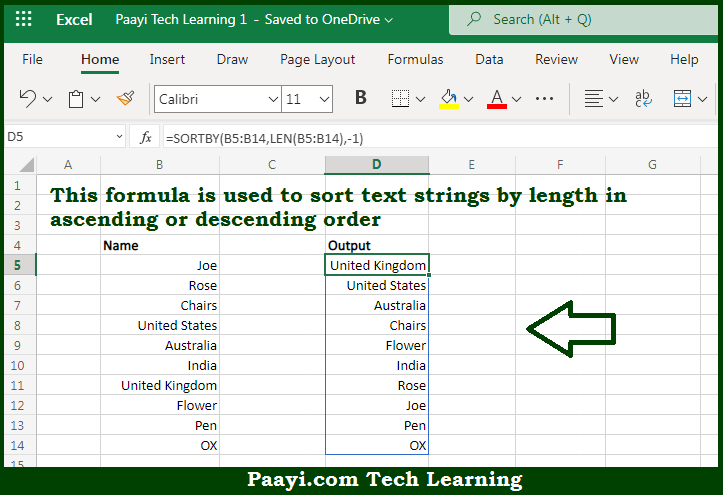# Learn How to Sort by Text Length in Microsoft Excel

Written by | 0 Comments | 599 Views

In this article, you will learn how to evaluate things in Dynamic Arrays with formulas in Microsoft Excel using a single/combination(s) of functions. You will also know How to Sort by Text Length and see the generic formula.

Learn How to Sort by Text Length in Microsoft Excel

The main purpose of this formula is to sort text strings by the length in ascending or descending order. Here we will learn how to sort by text length in the given data range in the workbook in Microsoft Excel. That implies, with the help of a formula based on the SORTBY, and LEN functions you can able to sort text strings by a length in ascending or descending order. So, with the help of this formula, you can able to sort by text length in the given data range in the workbook in Microsoft Excel.

General Formula to Sort by Text Length

=SORTBY(data,LEN(data),-1)

The Explanation to Sort by Text LengthSo we know that with the help of the given formula above you can able to sort text strings by a length in ascending or descending order. Here we will learn how to sort by text length in the given data range in the workbook in Microsoft Excel. As we know that the SORTBY function allows the sorting based on one or more "sort by" arrays, as long as dimensions are compatible with the source data. In the example given here, our goal is to sort the values in the B column by the number of characters each string contains. When working from the inside out, we use the LEN function to get the length of each value. So, with the help of this formula, you can able to sort text strings by the length in ascending or descending order. So now you have learned how to sort by text length, in the given data range in the workbook in Microsoft Excel.